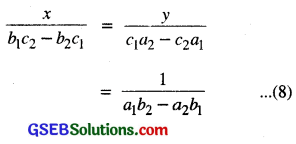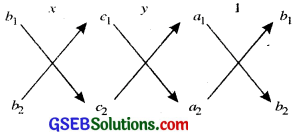# GSEB Class 10 Maths Notes Chapter 3 Pair of Linear Equations in Two Variables

This GSEB Class 10 Maths Notes Chapter 3 Pair of Linear Equations in Two Variables covers all the important topics and concepts as mentioned in the chapter.

## Pair of Linear Equations in Two Variables Class 10 GSEB Notes

We have learnt about linear equations in one variable and two variables.

The general form of a linear equation in two variables is ax + by + c = 0
or ax + by = c where a, b, c are real numbers and a ≠ 0, b ≠ 0.

Any pair of values of A and y which satisfies the equation ax + by + c = 0 or ax + by = c is called its solution. The graph of a linear equation in one variable is a straight line parallel to x-ax is or y-axis according as the equation is of the form ay = b or ax = b where a ≠ 0

The graph of a linear equation in two variables is also a straight line.
Every solution of linear equation determine on the line represented by it.

Pair of Linear Equations in Two Variables
Two linear equations which are in the same two variables x and y simultaneously are called a pair of linear equations in two variables.
The general form for a pair of linear equations in two variables x and y is
a1x + b1y + c1 = 0
and a2x + b2y + c2 = 0
where a1, b1, c1, a2, b2, c2 are all real numbers and a12 + b12 ≠ 0, a22 + b22 ≠ 0.

Solution Set: Solution set is collection of all those values of variables A and y satisfying each one of the equations in a given system of pair of linear equations in two variables.

Graphical Method of Solution of a Pair of Linear Equations
The behaviour of lines representing a pair of linear equations in two variables and the existence of solutions as follows:

• the lines may intersect in a single point. In this case, the pair of equations has a unique solution (consistent pair of equations).
• the lines may be parallel. In this case the equations have no solution (inconsistent pair of equations).
• the lines may be coincident. In this case, the equations have infinitely many solutions (dependent pair of equations).

The General equation of lines are represented by
a1x + b1y + c1 = 0
and a2x + b2y + c2 = 0
are

• intersecting, then $$\frac{a_{1}}{a_{2}} \neq \frac{b_{1}}{b_{2}}$$
• coincident, then $$\frac{a_{1}}{a_{2}}=\frac{b_{1}}{b_{2}}=\frac{c_{1}}{c_{2}}$$
• parallel, then $$\frac{a_{1}}{a_{2}}=\frac{b_{1}}{b_{2}} \neq \frac{c_{1}}{c_{2}}$$
The converse is also true for any pair of lines.

Algebraic Methods of Solving Pair of Linear Equations
In the previous section, we discussed how to solve a pair of linear equations graphically. The graphical method is not convenient in cases when the point representing the solution of the linear equations has non-integral coordinates. There is every possibility of making mistakes while reading such coordinates. There are several algebraic methods, which we shall now discuss.

Substitution Method: We shall explain die method of substitution by following steps ;
Step 1: Find the value of one variable, say y in terms of the other variable, i.e., x from either equation, whichever is convenient.

Step 2: Substitute this value of y in the other equation, and reduce it to an equation in one variable, i.e. in terms of x. which can be solved. Sometimes you can get equations with no variable. If this equation is true, you can conclude that the pair of linear equations has infinitely many solutions. If the equation in Step 2 is false, then the pair of linear equations is inconsistent.

Step 3: Substitute the value of x (or y) obtained in Step 2 in the equation used in Step 1 to obtain the value of the other variable.
Remark: We have substituted the value of one variable by expressing it in terms of other variable to solve the pair of linear equations. That is why the method is known as the substitution method.Elimination Method
In this method, we eliminate or remove one variable. This is sometimes more convenient than the substitution method.
In this method, we use the following steps to solve the equations:
Step 1: First multiply both the equations by some suitable non-zero constants to make the coefficients of one variable (either x or y) numerically equal.

Step 2: Then add or subtract one equation from the other so that one variable gets eliminated. If you get an equation in one variable, go to Step 3.

• If in Step 2, we obtain a true-equation involving no variable, then the original pair of equations has infinitely many solutions.
• If in Step 2, we obtain a false equation involving no variable, then the original pair of equations has no solution, i.e., it is inconsistent.

Step 3: Solve the equation in one variable (x or y) so obtained to get its value.

Step 4: Substitute this value of x (or y) in either of the original equations to get the value of the other variably.

Cross Multiplication Method
This method works for any pair of linear equations in two variables of the form
a1x + b1y + c1 = 0 …(1)
and a2x + b2y + c2 = 0 …(2)
To obtain the values of x and y as Shown above, we follow the following steps:
Step 1: Multiply equation (1) by b2 and equation (2) by b1 to get:
b2a1x + b2b1y + b2c1 = 0 …(3)
b1a2x + b1b2y + b1c2 = 0 …(4)

Step 2: Subtracting equation (4) and (3), we get:
(b2a1 – b1a2)x + (b2b1 – b1b2) y + (b2c1 – b1c2) = 0
(b2a1 – b1a2) x = b1c2 – b2c1
So, x = $$\frac{b_{1} c_{2}-b_{2} c_{1}}{a_{1} b_{2}-a_{2} b_{1}}$$
provided a1b2 – a2b1 ≠ 0 …(5)

Step 3: Substituting this value of x in (1) or (2), we get:
y = $$\frac{c_{1} a_{2}-c_{2} a_{1}}{a_{1} b_{2}-a_{2} b_{1}}$$ …………(6)
Now, two cases arise.
Case 1. a1b2 – a2b1 ≠ 0. In this case $$\frac{a_{1}}{a_{2}} \neq \frac{b_{1}}{b_{2}}$$. Then the pair of linear equations has a unique solution.

Case 2. a1b2 – a2b1 = 0. If we write $$\frac{a_{1}}{a_{2}}=\frac{b_{1}}{b_{2}}$$ = k then a1 = ka2, b1 = kb2.
Substituting the, values of a1 and b1 in the equation (1), we get:
k (a2x + b2y) + c1 =0……..(7)
It can be observed that the Equations (7) and (2) can both be satisfied only if c1 = k c2,
i-e., $$\frac{c_{1}}{c_{2}}$$ = k.

If c1 ≠ k c2, any solution of equation (2) will satisfy the equation (1), and vice versa. So, if
$$\frac{a_{1}}{a_{2}}=\frac{b_{1}}{b_{2}}=\frac{c_{1}}{c_{2}}$$ = k, then there are infinitely a2 b2 c2
many solutions to the pair of linear equations given by (1) and (2).

If c1 ≠ k c2, then any solution of equation (1) will not satisfy equation (2) and vice versa. Therefore the pair has no solution.
We can summarise the discussion above for the pair of linear equations given by (1) and (2) as follows:

• When $$\frac{a_{1}}{a_{2}} \neq \frac{b_{1}}{b_{2}}$$ we get a unique solution.
• When $$\frac{a_{1}}{a_{2}}=\frac{b_{1}}{b_{2}}=\frac{c_{1}}{c_{2}}$$ there are infinitely many solutions.
• When $$\frac{a_{1}}{a_{2}}=\frac{b_{1}}{b_{2}} \neq \frac{c_{1}}{c_{2}}$$ there is no solution.

Note that you can write the solution given by equations (5) and (6), in the following form:In remembering the above result, the following diagram may be helpful to you:The arrows between the two numbers indicate that they are to be multiplied and the second product is to be subtracted from the first.

For solving a pair of linear equations by this method we will follow the following steps:

• Step 1: Write the given equations in the form (1) and (2).
• Step 2: Taking the help of the diagram above, write equations as given in (8).
• Step 3: Findx and y, provided [a1b2 – a2b1] ≠ 0 Step 2 above gives you an indication of why this method is called the cross-multiplication method.

Equations Reducible to a Pair of Linear Equations in two Variables.
In this section, we shall discuss the solution of such pairs of equations which are not linear but can be reduced to linear form by making some suitable substitutions.